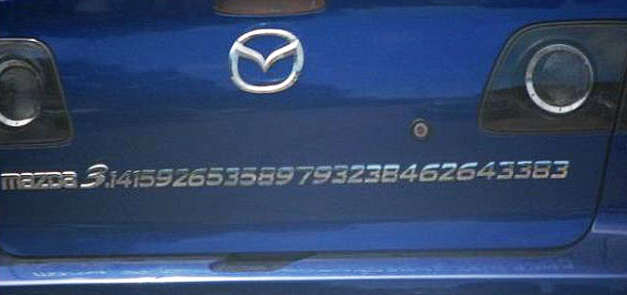## Welcome to H3 Maths

Blog Support for Growing Mathematicians

### The Mazda Pi – taking mathematics to a highway near you!

November28

I grew up with Mazda cars and loved the fact that they were so well designed by engineers – which is why most work could be done with just an 8mm and 10mm spanner! Since then cars have become more complicated, with important drive functions controlled by computers. So, why not extend the old Mazda models with a little mathematical pizzaz? Here is a good Californian example;by posted under Uncategorized | 1 Comment »
One Comment to

#### “The Mazda Pi – taking mathematics to a highway near you!”

1. T Says:

This reminded me of one of my friends! He has memorised Pi to 40 decimal places using a tune off of the IT crowd!

#### Post Support

NCEA Level 2 Algebra Problem. Using the information given, the shaded area = 9, that is:
y(y-8) = 9 –> y.y – 8y – 9 =0
–> (y-9)(y+1) = 0, therefore y = 9 (can’t have a distance of – 1 for the other solution for y)
Using the top and bottom of the rectangle,
x = (y-8)(y+2) = (9-8)(9+2) = 11
but, the left side = (x-4) = 11-4 = 7, but rhs = y+? = 9+?, which is greater than the value of the opp. side??
[I think that the left had side was a mistake and should have read (x+4)?]